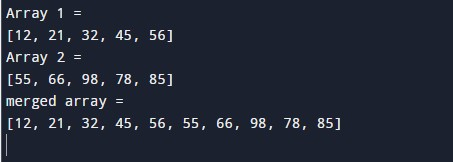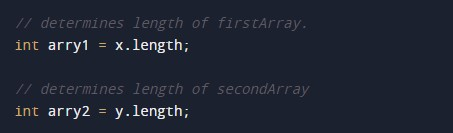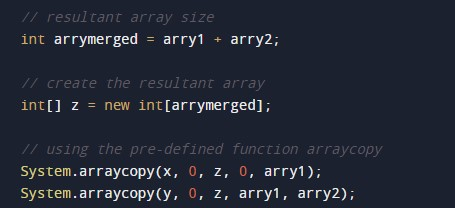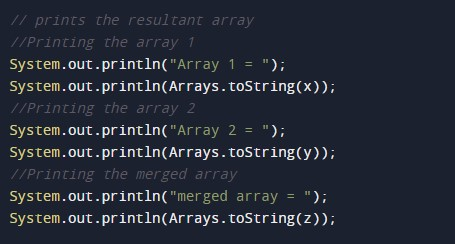# Java Program to Merge Two Sorted Arrays

In this tutorial you will learn about the Java Program to Merge Two Sorted Arrays and its application with practical example.

## Java Program to Merge Two Sorted Arrays

In this tutorial, we will learn to create a Java program that will Merge Two sorted Arrays using Java programming.

## Prerequisites

Before starting with this tutorial, we assume that you are the best aware of the following Java programming topics:

• Operators in Java Programming.
• Basic Input and Output function in Java Programming.
• Basic Java programming.
• For loop in Java programming.
• Arithmetic operations in Java Programming.

## Merging Two Arrays.

In this program, First, we will declare arrays one and two for the program. Then we will find the combined length for the merged array. After that, we will merge both the array. Then we will print the elements of the merged two sorted arrays to the user with the help of the print function.

With the help of this program, we can merge Two Sorted Arrays in an Array.

## Output:-In the above program, we have first initialized the required variable.• x = it will hold the integer value.
• y = it will hold the integer value.
• z = it will hold the integer value.

Finding the size of the arrays.Program Code to Merge Two Arrays.Printing output of the program.In this tutorial we have learn about the Java Program to Merge Two Sorted Arrays and its application with practical example. I hope you will like this tutorial.